Do you like the article?
Share it with others -

Use new possibilities of MetaTrader 5

# MQL5 Cookbook - Trading signals of moving channels

13 September 2016, 14:47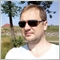1
17 079

### Introduction

The previous article «MQL5 Cookbook - Programming moving channels» described a method for plotting equidistant channels, frequently named as moving channels. In order to solve the task, the «Equidistant Channel» tool and OOP capabilities had been used.

This article will focus on signals, which can be identified by using these channels. Let us try to create a trading strategy based on these signals.

There are multiple articles published on MQL5, which describe generation of trading signals using calls to the ready modules of the Standard Library. Hopefully, this article will complement the materials and widen the users range of the standard classes.

Those starting to get acquainted with this strategy are welcome to learn the material from simple to complex. First, create a basic strategy, then make it more complex and add to it when possible.

### 1. The equidistant channels indicator

In the previous article on moving channels, the expert advisor plotted channels itself by creating graphical objects. On one hand, this approach facilitated the task for programmer, but on the other hand, rendered certain things impossible. For example, if the EA works in optimization mode, then it cannot detect any graphical objects on the chart, as there will be no chart at all. According to the limitations during testing:

Graphical Objects in Testing

During testing/optimization graphical objects are not plotted. Thus, when referring to the properties of a created object during testing/optimization, an Expert Advisor will receive zero values.

 This limitation does not apply to testing in visual mode.

Therefore, a different approach will be used, creating an indicator that reflects both fractals and the actual channel.

This indicator is called EquidistantChannels. It essentially consists of two blocks. The first calculates the fractal buffers, and the second — channel buffers.

Here is the code of the Calculate event handler.

```//+------------------------------------------------------------------+
//| Custom indicator iteration function                              |
//+------------------------------------------------------------------+
int OnCalculate(const int rates_total,
const int prev_calculated,
const datetime &time[],
const double &open[],
const double &high[],
const double &low[],
const double &close[],
const long &tick_volume[],
const long &volume[],
{
//--- if there were no bars at the previous call
if(prev_calculated==0)
{
//--- zero out the buffers
ArrayInitialize(gUpFractalsBuffer,0.);
ArrayInitialize(gDnFractalsBuffer,0.);
ArrayInitialize(gUpperBuffer,0.);
ArrayInitialize(gLowerBuffer,0.);
ArrayInitialize(gNewChannelBuffer,0.);
}
//--- Calculation for fractals [start]
int startBar,lastBar;
//---
if(rates_total<gMinRequiredBars)
{
Print("Not enough data for calculation");
return 0;
}
//---
if(prev_calculated<gMinRequiredBars)
startBar=gLeftSide;
else
startBar=rates_total-gMinRequiredBars;
//---
lastBar=rates_total-gRightSide;
for(int bar_idx=startBar; bar_idx<lastBar && !IsStopped(); bar_idx++)
{
//---
if(isUpFractal(bar_idx,gMaxSide,high))
gUpFractalsBuffer[bar_idx]=high[bar_idx];
else
gUpFractalsBuffer[bar_idx]=0.0;
//---
if(isDnFractal(bar_idx,gMaxSide,low))
gDnFractalsBuffer[bar_idx]=low[bar_idx];
else
gDnFractalsBuffer[bar_idx]=0.0;
}
//--- Calculation for fractals [end]

//--- Calculation for channel borders [start]
if(prev_calculated>0)
{
//--- if the set had not been initialized
if(!gFracSet.IsInit())
if(!gFracSet.Init(
InpPrevFracNum,
InpBarsBeside,
InpBarsBetween,
InpRelevantPoint,
InpLineWidth,
InpToLog
))
{
Print("Fractal set initialization error!");
return 0;
}
//--- calculation
gFracSet.Calculate(gUpFractalsBuffer,gDnFractalsBuffer,time,
gUpperBuffer,gLowerBuffer,
gNewChannelBuffer
);
}
//--- Calculation for channel borders [end]

//--- return value of prev_calculated for next call
return rates_total;
}
```

The block with the calculation of the fractal buffer values is highlighted in yellow, and the block with the calculation of channel buffers — in green. It is easy to notice that the second block will be activated not at the first, but only at the next call of the handler. This implementation of the second block allows to get filled fractal buffers.

Now, a couple of words about the set of fractal points — the CFractalSet object. Due to the change in the channel display method, it was also necessary to modify the CFractalSet class. The key method was the CFractalSet::Calculate, which calculates the channel buffer of the indicator. The code is provided in the CFractalPoint.mqh file.

Now there is a basis — provider of signals from the equidistant channel. Operation of the indicator is displayed in the video.

### 2. Basic strategy

So, let us start with something simple that can be improved and revised with the help of the OOP. Let there be some basic strategy.

This strategy will consider fairly simple trading rules. Market entries will be made by the channel borders. When the price touches the lower border a buy position will be opened, when it touches the lower border - a sell position. Fig. 1 shows that the price touched the lower border, so the robot bought a certain volume. The trade levels (stop loss and take profit) have a fixed size and were placed automatically. If there is position opened, the repeated entry signals will be ignored.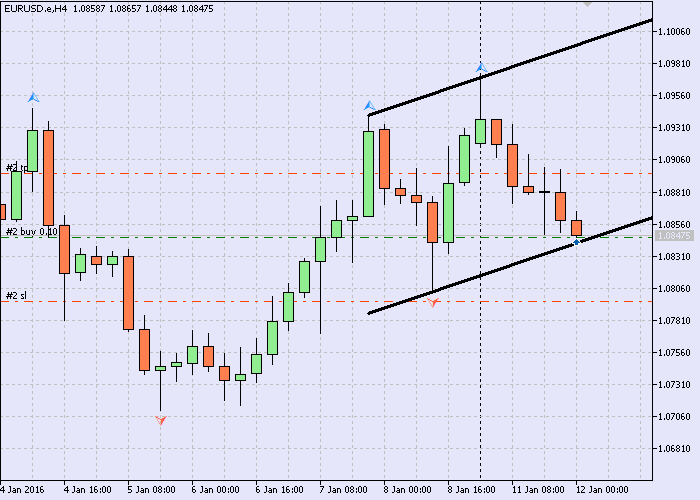Fig.1 Entry signal

It is also worth mentioning that the Standard library has grown quite a lot. It already contains many ready-made classes that can be used. First, let us try to «connect» to the signal class CExpertSignal. According to the documentation, it is base class for creating trading signal generators.

This class has been named quite accurately. This is not CTradeSignal and not CSignal, but namely the class of signals that are designed for use in the EA code — CExpertSignal.

I will not dwell on its content. The article «MQL5 Wizard: How to Create a Module of Trading Signals» contains a detailed description of the methods of the signal class.

2.1 The CSignalEquidChannel signal class

So, the derived signal class is as follows:

```//+------------------------------------------------------------------+
//| Class CSignalEquidChannel                                        |
//| Purpose: Class of trading signals based on equidistant           |
//|          channel.                                                |
//| Derived from the CExpertSignal class.                            |
//+------------------------------------------------------------------+
class CSignalEquidChannel : public CExpertSignal
{
protected:
CiCustom          m_equi_chs;          // indicator object "EquidistantChannels"
int               m_prev_frac_num;     // previous fractals
bool              m_to_plot_fracs;     // display fractals?
int               m_bars_beside;       // bars on the left/right of fractal
int               m_bars_between;      // intermediate bars
ENUM_RELEVANT_EXTREMUM m_relevant_pnt; // relevant point
int               m_line_width;        // line width
bool              m_to_log;            // keep the log?
double            m_pnt_in;            // internal tolerance, pips
double            m_pnt_out;           // external tolerance, pips
bool              m_on_start;          // signal flag on start
//--- calculated
double            m_base_low_price;    // base low price
double            m_base_high_price;   // base high price
double            m_upper_zone;     // upper zone: -internal tolerance, -external
double            m_lower_zone;     // lower zone
datetime          m_last_ch_time;      // occurrence time of the last channel
//--- "weights" of market models (0-100)
int               m_pattern_0;         //  "touching the lower border of the channel - buy, the upper - sell"

//--- === Methods === ---
public:
//--- Constructor/destructor
void              CSignalEquidChannel(void);
void             ~CSignalEquidChannel(void){};
//--- methods of setting adjustable parameters
void              PrevFracNum(int _prev_frac_num)   {m_prev_frac_num=_prev_frac_num;}
void              ToPlotFracs(bool _to_plot)        {m_to_plot_fracs=_to_plot;}
void              BarsBeside(int _bars_beside)      {m_bars_beside=_bars_beside;}
void              BarsBetween(int _bars_between)    {m_bars_between=_bars_between;}
void              RelevantPoint(ENUM_RELEVANT_EXTREMUM _pnt) {m_relevant_pnt=_pnt;}
void              LineWidth(int _line_wid)          {m_line_width=_line_wid;}
void              ToLog(bool _to_log)               {m_to_log=_to_log;}
void              PointsOutside(double _out_pnt)    {m_pnt_out=_out_pnt;}
void              PointsInside(double _in_pnt)      {m_pnt_in=_in_pnt;}
void              SignalOnStart(bool _on_start)     {m_on_start=_on_start;}
//--- methods of adjusting "weights" of market models
void              Pattern_0(int _val) {m_pattern_0=_val;}
//--- method of verification of settings
virtual bool      ValidationSettings(void);
//--- method of creating the indicator and time series
virtual bool      InitIndicators(CIndicators *indicators);
//--- methods of checking if the market models are generated
virtual int       LongCondition(void);
virtual int       ShortCondition(void);
virtual double    Direction(void);
//---
protected:
//--- method of initialization of the indicator
bool              InitCustomIndicator(CIndicators *indicators);
//- get the value of the upper border of the channel
double            Upper(int ind) {return(m_equi_chs.GetData(2,ind));}
//- get the value of the lower border of the channel
double            Lower(int ind) {return(m_equi_chs.GetData(3,ind));}
//- get the flag of channel occurrence
double            NewChannel(int ind) {return(m_equi_chs.GetData(4,ind));}
};
//+------------------------------------------------------------------+
```

A few nuances to be noted.

The main signal generator in this class is the equidistant channel setup. And it is the only one in the current version. For now, there will not be any others at all. In its turn, this class contains a class for working with technical indicator of a custom typeCiCustom.

The basic model is used as the signal model: "touching the lower border of the channel — buy, the upper — sell". Since touching with pinpoint precision is, so to say, not the most probable event, it uses a certain buffer with adjustable borders. The external tolerance parameter m_pnt_out determines how far the price is allowed to go beyond the channel borders, and the internal tolerance parameter m_pnt_in — how far the price is allowed to stay away from the border. The logic is quite simple. Assume that the price touched the channel border if it came very close to it or slightly exceeded it. Fig.2 schematically shows a buffer. When price enters it from below, the price with the border triggers the model.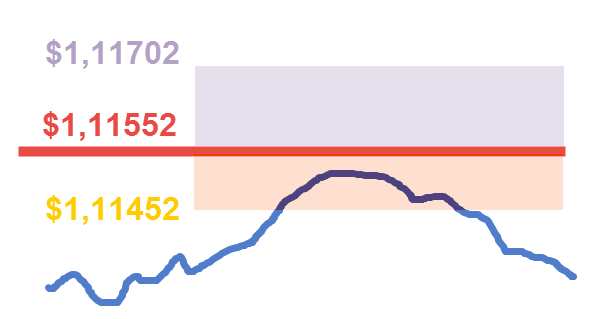Fig.2 Triggering the basic signal model

The m_upper_zone parameter array outlines the borders of the upper buffer, and the m_lower_zone — the lower.

The level at \$1,11552 in the example serves as the upper border of the channel (red line). The level at \$1,11452 is responsible for the lower limit of the buffer, and \$1,11702 — for the upper. Thus, the size of the external tolerance is 150 points, and the internal is 100 points. The price is displayed by the blue curve.

The m_on_start parameter allows to ignore the signals of the first channel when running the robot on the chart, in case such a channel had been drawn already. If the flag is reset, the robot will work only on the next channel and will not process trading signals on the current one.

The m_base_low_price and m_base_high_price parameters store the values of the low and high prices of the actual bar. That is considered to be the zero bar if the trading is performed on every tick, or the previous bar if trading is allowed only at the appearance of a new bar.

Now, a few words about the methods. It should be noted here that the developer provides a sufficiently broad freedom of action, as about half of the methods are virtual. And this means that the behavior of the descendant classes can be implemented as necessary.

```//+------------------------------------------------------------------+
//| Determining the "weighted" direction                             |
//+------------------------------------------------------------------+
double CSignalEquidChannel::Direction(void)
{
double result=0.;
//--- appearance of a new channel
datetime last_bar_time=this.Time(0);
bool is_new_channel=(this.NewChannel(0)>0.);
//--- if the signals of the first channel are ignored
if(!m_on_start)
//--- if the first channel is usually displayed during initialization
if(m_prev_frac_num==3)
{
static datetime last_ch_time=0;
//--- if a new channel appeared
if(is_new_channel)
{
last_ch_time=last_bar_time;
//--- if this is the first launch
if(m_last_ch_time==0)
//--- store the time of the bar where the first channel had appeared
m_last_ch_time=last_ch_time;
}
//--- if the times match
if(m_last_ch_time==last_ch_time)
return 0.;
else
//--- clear the flag
m_on_start=true;
}
//--- index of the actual bar
int actual_bar_idx=this.StartIndex();
//--- set the borders
double upper_vals,lower_vals; // -bar preceding the actual, -actual bar
ArrayInitialize(upper_vals,0.);
ArrayInitialize(lower_vals,0.);
for(int idx=ArraySize(upper_vals)-1,jdx=0;idx>=0;idx--,jdx++)
{
upper_vals[jdx]=this.Upper(actual_bar_idx+idx);
lower_vals[jdx]=this.Lower(actual_bar_idx+idx);
if((upper_vals[jdx]==0.) || (lower_vals[jdx]==0.))
return 0.;
}
//--- get the prices
double curr_high_pr,curr_low_pr;
curr_high_pr=this.High(actual_bar_idx);
curr_low_pr=this.Low(actual_bar_idx);
//--- if the prices are obtained
if(curr_high_pr!=EMPTY_VALUE)
if(curr_low_pr!=EMPTY_VALUE)
{
//--- store the prices
m_base_low_price=curr_low_pr;
m_base_high_price=curr_high_pr;
//--- Define prices for buffer zones
//--- upper zone: -internal tolerance, -external
this.m_upper_zone=upper_vals-m_pnt_in;
this.m_upper_zone=upper_vals+m_pnt_out;
//--- lower zone: -internal tolerance, -external
this.m_lower_zone=lower_vals+m_pnt_in;
this.m_lower_zone=lower_vals-m_pnt_out;
//--- normalization
for(int jdx=0;jdx<ArraySize(m_lower_zone);jdx++)
{
this.m_lower_zone[jdx]=m_symbol.NormalizePrice(m_lower_zone[jdx]);
this.m_upper_zone[jdx]=m_symbol.NormalizePrice(m_upper_zone[jdx]);
}
//--- check if the zones converge
if(this.m_upper_zone<=this.m_lower_zone)
return 0.;
//--- Result
result=m_weight*(this.LongCondition()-this.ShortCondition());
}
//---
return result;
}
//+------------------------------------------------------------------+
```

The first block in the body of the method checks whether it is necessary to ignore the first channel on the chart, if such is present.

The second block obtains the current prices and determines the buffer zones. This is the check for convergence of the zones. If the channel is too narrow or the buffer zones too wide, there is a purely mathematical possibility that the price may go into both zones. Therefore, such situation should be handled.

The target line is highlighted in blue. Here, it gets a quantitative estimate of the trading direction, if there is any.

Now, let us consider the LongCondition() method.

```//+------------------------------------------------------------------+
//| Check condition for buying                                       |
//+------------------------------------------------------------------+
int CSignalEquidChannel::LongCondition(void)
{
int result=0;
//--- if the low price is set
if(m_base_low_price>0.)
//--- if the low price is at the level of the lower border
if((m_base_low_price<=m_lower_zone) && (m_base_low_price>=m_lower_zone))
{
if(IS_PATTERN_USAGE(0))
result=m_pattern_0;
}
//---
return result;
}
//+------------------------------------------------------------------+
```

For buying, check if the price got into the lower buffer zone. If it did, check the permission for activating the market model. More details on structures of type "IS_PATTERN_USAGE(k)" can be found in the article

The ShortCondition() method works similarly to the above. Only the focus is on the upper buffer zone.

```//+------------------------------------------------------------------+
//| Check condition for selling                                      |
//+------------------------------------------------------------------+
int CSignalEquidistantChannel::ShortCondition(void)
{
int result=0;
//--- if the high price is set
if(m_base_high_price>0.)
//--- if the high price is at the level of the upper border
if((m_base_high_price>=m_upper_zone) && (m_base_high_price<=m_upper_zone))
{
if(IS_PATTERN_USAGE(0))
result=m_pattern_0;
}
//---
return result;
}
//+------------------------------------------------------------------+
```

The class initializes a custom indicator using the InitCustomIndicator() method:

```//+------------------------------------------------------------------+
//| Initialization of custom indicators                              |
//+------------------------------------------------------------------+
bool CSignalEquidChannel::InitCustomIndicator(CIndicators *indicators)
{
//--- add an object to the collection
{
return false;
}
//--- specifies indicator parameters
MqlParam parameters;
parameters.type=TYPE_STRING;
parameters.string_value="EquidistantChannels.ex5";
parameters.type=TYPE_INT;
parameters.integer_value=m_prev_frac_num;   // 1) previous fractals
parameters.type=TYPE_BOOL;
parameters.integer_value=m_to_plot_fracs;   // 2) display fractals?
parameters.type=TYPE_INT;
parameters.integer_value=m_bars_beside;     // 3) bars on the left/right of fractal
parameters.type=TYPE_INT;
parameters.integer_value=m_bars_between;    // 4) intermediate bars
parameters.type=TYPE_INT;
parameters.integer_value=m_relevant_pnt;    // 5) relevant point
parameters.type=TYPE_INT;
parameters.integer_value=m_line_width;    // 6) line width
parameters.type=TYPE_BOOL;
parameters.integer_value=m_to_log;          // 7) keep the log?

//--- object initialization
if(!m_equi_chs.Create(m_symbol.Name(),_Period,IND_CUSTOM,8,parameters))
{
PrintFormat(__FUNCTION__+": error initializing object");
return false;
}
//--- number of buffers
if(!m_equi_chs.NumBuffers(5))
return false;
//--- ok
return true;
}
//+------------------------------------------------------------------+
```

The first value in the parameter array should be the indicator name as a string.

The class also contains a virtual ValidationSettings() method. It calls a similar method of the ancestor and checks if the parameters of the channel indicator had been set correctly. There are also service methods that get the values of the corresponding buffers of the custom indicator.

For now, this is everything related to the derived signal class.

Implementation of the basic idea will require writing a class derived from the CExpert standard class. At the current step, the code will be as compact as possible, because, in fact, it is necessary to change only the behavior of the main handler — the Processing() method. It is virtual, which grants the opportunity to write any strategies.

```//+------------------------------------------------------------------+
//| Class CEquidChannelExpert.                                       |
//| Purpose: Class for EA that trades based on equidistant channel.  |
//| Derived from the CExper class.                                   |
//+------------------------------------------------------------------+
class CEquidChannelExpert : public CExpert
{
//--- === Data members === ---
private:

//--- === Methods === ---
public:
//--- constructor/destructor
void              CEquidChannelExpert(void){};
void             ~CEquidChannelExpert(void){};

protected:
virtual bool      Processing(void);
};
//+------------------------------------------------------------------+
```

Here is the method itself:

```//+------------------------------------------------------------------+
//| Main module                                                      |
//+------------------------------------------------------------------+
bool CEquidChannelExpert::Processing(void)
{
//--- calculation of the direction
m_signal.SetDirection();
//--- check if open positions
if(!this.SelectPosition())
{
//--- position opening module
if(this.CheckOpen())
return true;
}
//--- if there are no trade operations
return false;
}
```

Everything is quite simple. First, the signal object estimates the possible trading direction, after that the presence of an open position is checked. If there is no position, it looks for an opportunity to open it. If there is a position, then leave.

The code of the basic strategy is implemented in the BaseChannelsTrader.mq5 file.

Example of the basic strategy operation is presented in the video.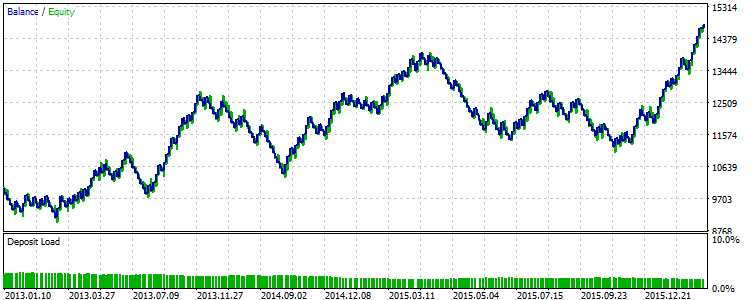Fig.3 Results of the basic strategy for 2013-2015.

The run was made in the strategy tester on the hourly timeframe for the EURUSD symbol. It can be noticed on the balance chart that in certain intervals the basic strategy worked by the "saw principle": an unprofitable trade was followed by a profitable one. The custom parameter values used in testing are available in the base_signal.set file. It also contains the channel parameters, the values of which will remain unchanged in all versions of the strategy.

Here and below, the "Every tick based on real ticks" testing mode is used.

Essentially, there are 2 ways to improve the trading performance of the strategy. The first is optimization, which lies in selecting such a combination of parameter values that would maximize profit, etc. The second way concerns finding the factors that affect the performance of the EA. If the first method is not associated with changing the logic of the trading strategy, the second one cannot do without it.

In the next section, the basic strategy will be edited and the performance factors will be sought.

### 3. Performance factors

A few words about the disposition. It may be convenient to place all files of the strategy that make it unique into a single project folder. Thus, implementation of the basic strategy is located in the Base subfolder (Fig.4) and so on.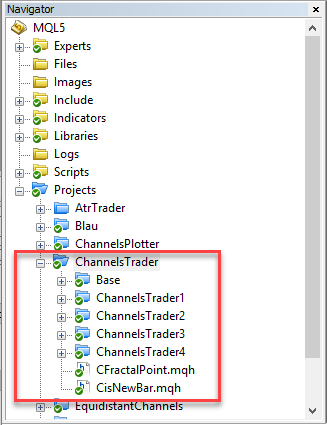Fig.4 Example of project folder hierarchy for the channels strategy

Further, assume that each new factor is a new stage for making changes to the source files that make up the EA code.

3.1 Using the trailing

Before starting, it is suggested to add the trailing feature to the strategy. Let it be an object of the CTrailingFixedPips class, which allows to maintain open positions at a fixed "distance" (in points). This will trail both the stop loss price and the take profit price. To disable trailing the take profit, set a zero value to the corresponding parameter (InpProfitLevelPips).

Make the following changes in the code:

Add a group of custom parameters to the ChannelsTrader1.mq5 file of the expert:

```//---
sinput string Info_trailing="+===-- Trailing --====+"; // +===-- Trailing --====+
input int InpStopLevelPips=30;          // Level for StopLoss, pips
input int InpProfitLevelPips=50;        // Level for TakeProfit, pips
```

In the initialization block, write that an object of CTrailingFixedPips type is to be created, include it in the strategy and set the trailing parameters.

```//--- trailing object
CTrailingFixedPips *trailing=new CTrailingFixedPips;
if(trailing==NULL)
{
//--- error
printf(__FUNCTION__+": error creating trailing");
myChannelExpert.Deinit();
return(INIT_FAILED);
}
if(!myChannelExpert.InitTrailing(trailing))
{
//--- error
PrintFormat(__FUNCTION__+": error initializing trailing");
myChannelExpert.Deinit();
return INIT_FAILED;
}
//--- trailing parameters
trailing.StopLevel(InpStopLevelPips);
trailing.ProfitLevel(InpProfitLevelPips);
```

Since trailing will be used, it is necessary to modify the main CEquidChannelExpert::Processing() method in the EquidistantChannelExpert1.mqh file as well.

```//+------------------------------------------------------------------+
//| Main module                                                      |
//+------------------------------------------------------------------+
bool CEquidChannelExpert::Processing(void)
{
//--- calculation of the direction
m_signal.SetDirection();
//--- if there is no position
if(!this.SelectPosition())
{
//--- position opening module
if(this.CheckOpen())
return true;
}
//--- if the position exists
else
{
//--- checking if position modification is possible
if(this.CheckTrailingStop())
return true;
}
//--- if there are no trade operations
return false;
}
```

That's it. Trailing has been added. Files of the updated strategy are located in a separate ChannelsTrader1 subfolder.

Let us check if the innovation has any impact on effectiveness.

So, multiple runs in optimization mode have been made in the strategy tester, with the same history interval and parameter values as for the basic strategy. The stop loss and take profit parameters have been adjusted:

Variable StartStep
Stop
Level for StopLoss, pips
0
10
100
Level for TakeProfit, pips
0
10
150

The optimization results can be found in the ReportOptimizer-signal1.xml file. The best run is presented in Fig.5, where the level for StopLoss = 0, and for TakeProfit = 150.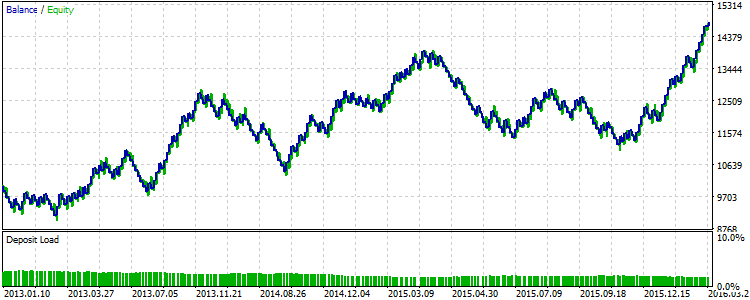Fig.5 Results of the strategy with the use of trailing for 2013-2015.

It is easy to notice that the last figure resembles Fig 3. Thus it can be said that the use of trailing in this value range did not improve the outcome.

3.2 Channel type

There is an assumption that the channel type affects the performance results. The general idea is this: it is better to sell in a descending channel, and to buy in ascending. If the channel is flat (not inclined), then it is possible to trade based on both borders.

The ENUM_CHANNEL_TYPE enumeration defines the channel type:

```//+------------------------------------------------------------------+
//| Channel type                                                     |
//+------------------------------------------------------------------+
enum ENUM_CHANNEL_TYPE
{
CHANNEL_TYPE_ASCENDING=0,  // ascending
CHANNEL_TYPE_DESCENDING=1, // descending
CHANNEL_TYPE_FLAT=2,       // flat
};
//+------------------------------------------------------------------+
```

Define the tolerance parameter to search for the channel type in the initialization block of the ChannelsTrader2.mq5 source file of the EA.

```//--- filter parameters
filter0.PointsInside(_Point*InpPipsInside);
filter0.PointsOutside(_Point*InpPipsOutside);
filter0.TypeTolerance(_Point*InpTypePips);
filter0.PrevFracNum(InpPrevFracNum);
...
```

This parameter controls the speed of price change in points. Assume that it is equal to 7 pips. Then, if the channel "grows" by 6 pips every bar, it is not enough to be considered ascending. Then it will simply be considered flat (not inclined).

Add the identification of the channel type to the Direction() method of the SignalEquidChannel2.mqh source signal of the signal.

```//--- if the channel is new
if(is_new_channel)
{
m_ch_type=CHANNEL_TYPE_FLAT;                // flat (not inclined) channel
//--- if tolerance for the type is set
if(m_ch_type_tol!=EMPTY_VALUE)
{
//--- Channel type
//--- speed of change
double pr_speed_pnt=m_symbol.NormalizePrice(upper_vals-upper_vals);
//--- if the speed is sufficient
if(MathAbs(pr_speed_pnt)>m_ch_type_tol)
{
if(pr_speed_pnt>0.)
m_ch_type=CHANNEL_TYPE_ASCENDING;  // ascending channel
else
m_ch_type=CHANNEL_TYPE_DESCENDING; // descending channel
}
}
}
```

Initially, the channel is considered to be flat - not ascending and not descending. If the value of the tolerance parameter for identifying the channel type had not been set, then it will not come to determining the speed of changing.

The condition for buying will include a check of that the channel is not descending.

```//+------------------------------------------------------------------+
//| Check condition for buying                                       |
//+------------------------------------------------------------------+
int CSignalEquidChannel::LongCondition(void)
{
int result=0;
//--- if the low price is set
if(m_base_low_price>0.)
//--- if the channel is not descending
if(m_ch_type!=CHANNEL_TYPE_DESCENDING)
//--- if the low price is at the level of the lower border
if((m_base_low_price<=m_lower_zone) && (m_base_low_price>=m_lower_zone))
{
if(IS_PATTERN_USAGE(0))
result=m_pattern_0;
}
//---
return result;
}
//+------------------------------------------------------------------+
```

A similar check is performed in the condition for selling to see if the channel is not ascending.

The main CEquidChannelExpert::Processing() method if the EquidistantChannelExpert2.mqh file will be the same as in the basic version, since trailing is excluded.

Check the effectiveness of this factor. Only one parameter is optimized.

Variable StartStep
Stop
Tolerance for type, pips
0
5
150

The optimization results can be found in the ReportOptimizer-signal2.xml file. The best run is presented in Fig.6.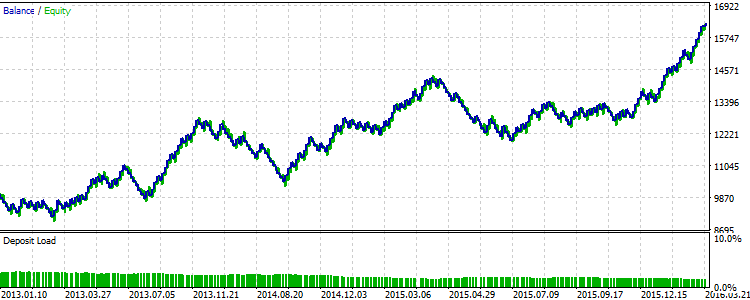Fig.6 Results of the strategy with the use of channel type for 2013-2015.

It is easy to notice that the strategy testing results are slightly better that the results of the basic strategy. It turns out that, at the given base value of parameters, a filter like channel type influences the final result.

3.3 Channel width

It seems that the channel width may influence the type of strategy itself. If the channel turned out narrow, then when its border is broken, it would be possible to trade towards the breakout direction and not against it. This results in a breakout strategy. If the channel turned out wide, it is possible to trade based on its borders. This is the rebound strategy. This is what the current strategy is — trading is performed based on the channel borders.

Obviously, a criterion is required here for determining if the channel is narrow or wide. In order not to go to extremes, it is suggested to add something in between, to consider the analyzed channel neither narrow nor wide. As a result, 2 criteria are required:

1. sufficient width of a narrow channel;
2. sufficient width of a wide channel.

If the channel is neither, then it may be wise to refrain from entering the market.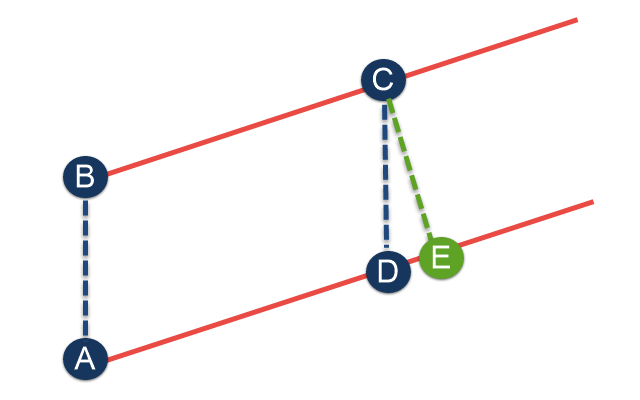Fig.7 Channel width, diagram

It should be noted that there is a geometric problem with determining the channel width. As the chart axes are measured in different values. Thus, it is easy to measure the length of the AB and CD segments. But there is a problem with the calculation of the CE segment (Fig.7).

The simplest method has been chosen for normalization, though perhaps controversial and not the most accurate one. The formula is as follows:

length of CE ≃ length of CD / (1.0 + channel speed)

Channel width is measured using the ENUM_CHANNEL_WIDTH_TYPE enumeration:

```//+------------------------------------------------------------------+
//| Channel width                                                    |
//+------------------------------------------------------------------+
enum ENUM_CHANNEL_WIDTH_TYPE
{
CHANNEL_WIDTH_NARROW=0,   // narrow
CHANNEL_WIDTH_MID=1,      // average
};
```

Add the channel width criteria to the group of "Channels" custom parameters to the ChannelsTrader3.mq5 expert source file.

```//---
sinput string Info_channels="+===-- Channels --====+"; // +===-- Channels --====+
input int InpPipsInside=100;            // Internal tolerance, pips
input int InpPipsOutside=150;           // External tolerance, pips
input int InpNarrowPips=250;            // Narrow channel, pips
input int InpBroadPips=1200;            // Wide channel, pips
...
```

If the criterion of the narrow channel has a value greater than that of the wide channel, an initialization error will take place.

```//--- filter parameters
filter0.PointsInside(_Point*InpPipsInside);
filter0.PointsOutside(_Point*InpPipsOutside);
{
PrintFormat(__FUNCTION__+": error specifying narrow and broad values");
return INIT_FAILED;
}
filter0.NarrowTolerance(_Point*InpNarrowPips);
```

The moment of determining the degree of channel width is presented in the body of the Direction() method.

```//--- Channel width
m_ch_width=CHANNEL_WIDTH_MID;               // average
double ch_width_pnt=((upper_vals-lower_vals)/(1.0+pr_speed_pnt));
//--- if the narrow channel criterion is specified
if(m_ch_narrow_tol!=EMPTY_VALUE)
if(ch_width_pnt<=m_ch_narrow_tol)
m_ch_width=CHANNEL_WIDTH_NARROW;      // narrow
//--- if the wide channel criterion is specified
if(m_ch_narrow_tol!=EMPTY_VALUE)
```

Initially, the channel is considered to be average. After that, it is checked if it is narrow or wide.

It is also necessary to change the methods for determining the trading direction as well. Thus, the condition for buying will look the following way:

```//+------------------------------------------------------------------+
//| Check condition for buying                                       |
//+------------------------------------------------------------------+
int CSignalEquidChannel::LongCondition(void)
{
int result=0;
//--- if the channel is narrow - trade the breakout of the upper border
if(m_ch_width==CHANNEL_WIDTH_NARROW)
{
//--- if the high price is set
if(m_base_high_price>0.)
//--- if the high price is at the level of the upper border
if(m_base_high_price>=m_upper_zone)
{
if(IS_PATTERN_USAGE(0))
result=m_pattern_0;
}
}
//--- or if the channel is wide - trade the rebound from the lower border
{
//--- if the low price is set
if(m_base_low_price>0.)
//--- if the low price is at the level of the lower border
if((m_base_low_price<=m_lower_zone) && (m_base_low_price>=m_lower_zone))
{
if(IS_PATTERN_USAGE(0))
result=m_pattern_0;
}
}
//---
return result;
}
//+------------------------------------------------------------------+
```

The method consists of two blocks. The first checks the opportunity to trade the breakout within the narrow channel. Note that in the current variant, the breakout is considered to be the price reaching to top of the upper buffer zone. The second block checks if the price had already got into the lower buffer zone for the rebound strategy to come into play.

The method of checking the opportunity to sell — ShortCondition() — is created by analogy.

The main CEquidChannelExpert::Processing() method in the EquidistantChannelExpert3.mqh file remains unchanged.

There are 2 parameters to be optimized.

Variable StartStep
Stop
Narrow channel, pips
100
20
250
Wide channel, pips
350
50
1250

The optimization results can be found in the ReportOptimizer-signal3.xml file. The best run is presented in Fig.8.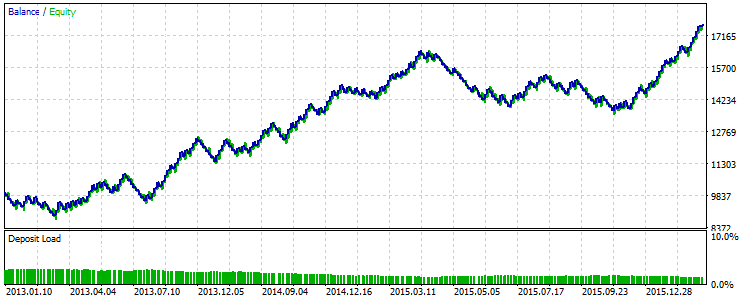Fig.8 Results of the strategy with the consideration of channel width for 2013-2015.

Perhaps, this is the factor with the most impact among all the described above. The balance curve now has a more pronounced direction.

3.4 Borderline stop loss and take profit levels

If the trade targets are originally present in the form of stop loss and take profit levels, then there should be the ability to adjust these levels to the conditions of the current strategy. Simply put, if there is a channel that makes its way through the dynamics on the chat at a certain angle, the stop loss and take profit levels should be moved in conjunction with the channel borders.

A couple of models have been added for convenience. Now they look like this:

```//--- "weights" of market models (0-100)
int               m_pattern_0;         //  "Rebound from channel border" model
int               m_pattern_1;         //  "Breakout of channel border" model
int               m_pattern_2;         //  "New channel" model
```

The previous versions had only one, and it was responsible for the price touching any border of the channel. Now, the rebound and breakout model will be differentiated. Now there is also the third model — new channel model. It is required for cases when there is a new channel and there is a position opened on the past channel. If the model was triggered, the position will be closed.

The condition for buying looks the following way:

```//+------------------------------------------------------------------+
//| Check condition for buying                                       |
//+------------------------------------------------------------------+
int CSignalEquidChannel::LongCondition(void)
{
int result=0;
bool is_position=PositionSelect(m_symbol.Name());
//--- if the channel is narrow - trade the breakout of the upper border
if(m_ch_width_type==CHANNEL_WIDTH_NARROW)
{
//--- if the high price is set
if(m_base_high_price>0.)
//--- if the high price is at the level of the upper border
if(m_base_high_price>=m_upper_zone)
{
if(IS_PATTERN_USAGE(1))
{
result=m_pattern_1;
//--- if there is no position
if(!is_position)
//--- to the Journal
if(m_to_log)
{
Print("\nTriggered the \"Breakout of channel border\" model for buying.");
PrintFormat("High price: %0."+IntegerToString(m_symbol.Digits())+"f",m_base_high_price);
PrintFormat("Trigger price: %0."+IntegerToString(m_symbol.Digits())+"f",m_upper_zone);
}
}
}
}
//--- or if the channel is wide or average - trade the rebound from the lower border
else
{
//--- if the low price is set
if(m_base_low_price>0.)
//--- if the low price is at the level of the lower border
if((m_base_low_price<=m_lower_zone) && (m_base_low_price>=m_lower_zone))
{
if(IS_PATTERN_USAGE(0))
{
result=m_pattern_0;
//--- if there is no position
if(!is_position)
//--- to the Journal
if(m_to_log)
{
Print("\nTriggered the \"Rebound of channel border\" model for buying.");
PrintFormat("Low price: %0."+IntegerToString(m_symbol.Digits())+"f",m_base_low_price);
PrintFormat("Zone up: %0."+IntegerToString(m_symbol.Digits())+"f",m_upper_zone);
PrintFormat("Zone down: %0."+IntegerToString(m_symbol.Digits())+"f",m_upper_zone);
}
}
}
}
//---
return result;
}
//+------------------------------------------------------------------+
```

Also, there is now a check of the condition for selling:

```//+------------------------------------------------------------------+
//| Check condition for closing a buy                                |
//+------------------------------------------------------------------+
bool CSignalEquidChannel::CheckCloseLong(double &price) const
{
bool to_close_long=true;
int result=0;
if(IS_PATTERN_USAGE(2))
result=m_pattern_2;
if(result>=m_threshold_close)
{
if(m_is_new_channel)
//--- if a buy is to be closed
if(to_close_long)
{
price=NormalizeDouble(m_symbol.Bid(),m_symbol.Digits());
//--- to the Journal
if(m_to_log)
{
Print("\nTriggered the \"New channel\" model for closing buy.");
PrintFormat("Close price: %0."+IntegerToString(m_symbol.Digits())+"f",price);
}
}
}
//---
}
//+------------------------------------------------------------------+
```
For a short position, the condition for closing will be identical.

Now, a few words about the trailing. A separate CTrailingEquidChannel class has been written for it, with the CExpertTrailing class being its parent.

```//+------------------------------------------------------------------+
//| Class CTrailingEquidChannel.                                     |
//| Purpose: Class of trailing stops based on Equidistant Channel.   |
//|              Derives from class CExpertTrailing.                 |
//+------------------------------------------------------------------+
class CTrailingEquidChannel : public CExpertTrailing
{
protected:
double            m_sl_distance;       // distance to stop loss
double            m_tp_distance;       // distance to take profit
double            m_upper_val;         // upper border
double            m_lower_val;         // lower border
ENUM_CHANNEL_WIDTH_TYPE m_ch_wid_type; // channel type by width
//---
public:
void              CTrailingEquidChannel(void);
void             ~CTrailingEquidChannel(void){};
//--- methods of initialization of protected data
//---
virtual bool      CheckTrailingStopLong(CPositionInfo *position,double &sl,double &tp);
virtual bool      CheckTrailingStopShort(CPositionInfo *position,double &sl,double &tp);
//---
bool              RefreshData(const CSignalEquidChannel *_ptr_ch_signal);
};
//+------------------------------------------------------------------+
```

The method for getting the information from the channel signal is highlighted in red.

The methods for checking the possibility of trailing for short and long positions of the ancestor have been redefined using polymorphism - the basic principle of the OOP.

For the trailing class to be able to receive the time and price targets of the actual channel, it was necessary to create a binding with the CSignalEquidChannel signal class. It was implemented in the constant pointer within the CEquidChannelExpert class. This approach allows to obtain all the necessary information from the signal, without the danger of changing the parameters of the signal itself.

```//+------------------------------------------------------------------+
//| Class CEquidChannelExpert.                                       |
//| Purpose: Class for EA that trades based on equidistant channel.  |
//| Derived from the CExper class.                                   |
//+------------------------------------------------------------------+
class CEquidChannelExpert : public CExpert
{
//--- === Data members === ---
private:
const CSignalEquidChannel *m_ptr_ch_signal;

//--- === Methods === ---
public:
//--- constructor/destructor
void              CEquidChannelExpert(void);
void             ~CEquidChannelExpert(void);
//--- pointer to the channel signal object
void              EquidChannelSignal(const CSignalEquidChannel *_ptr_ch_signal){m_ptr_ch_signal=_ptr_ch_signal;};
const CSignalEquidChannel *EquidChannelSignal(void) const {return m_ptr_ch_signal;};

protected:
virtual bool      Processing(void);
virtual bool      CheckClose(void);
virtual bool      CheckCloseLong(void);
virtual bool      CheckCloseShort(void);
//--- trailing stop check
virtual bool      CheckTrailingStop(void);
virtual bool      CheckTrailingStopLong(void);
virtual bool      CheckTrailingStopShort(void);
};
//+------------------------------------------------------------------+
```

The methods responsible for closure and trailing have also been redefined in the expert class.

The main CEquidChannelExpert::Processing() method in the EquidistantChannelExpert4.mqh file looks as follows:

```//+------------------------------------------------------------------+
//| Main module                                                      |
//+------------------------------------------------------------------+
bool CEquidChannelExpert::Processing(void)
{
//--- calculation of the direction
m_signal.SetDirection();
//--- if there is no position
if(!this.SelectPosition())
{
//--- position opening module
if(this.CheckOpen())
return true;
}
//--- if the position exists
else
{
if(!this.CheckClose())
{
//--- checking if position modification is possible
if(this.CheckTrailingStop())
return true;
//---
return false;
}
else
{
return true;
}
}
//--- if there are no trade operations
return false;
}
//+------------------------------------------------------------------+
```
These parameters will be optimized:
Variable StartStep
Stop
Stop loss, points
25
5
75
Take profit, points50
5
200

The optimization results can be found in the ReportOptimizer-signal4.xml file. The best run is presented in Fig.9.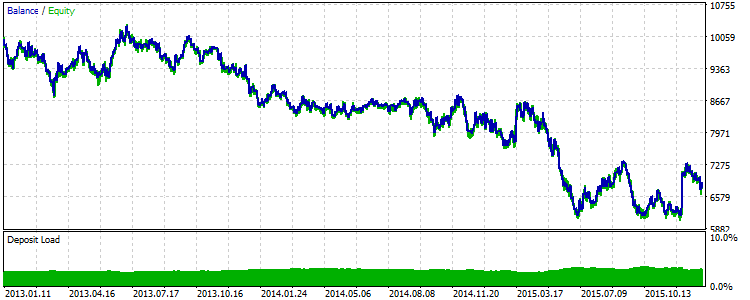Fig.9 Results of the strategy with the consideration of borderline levels for 2013-2015.

It is clear that this factor — borderline price levels — did not improve the performance.

### Conclusion

The article presented the process of developing and implementing a class for sending signals based on the moving channels. Each of the signal version was followed by a trading strategy with testing results.

It should be stressed that fixed values for equidistant channel settings have been used throughout the article. Therefore, the conclusions on whether one or another factor had been effective are true only for the specified values.

There still are other ways to improve the performance results. This article covered part of the work on finding such possibilities.

Translated from Russian by MetaQuotes Software Corp.
Original article: https://www.mql5.com/ru/articles/1863

Attached files |
reports.zip (18.06 KB)
base_signal.set (1.54 KB)
Last comments | Go to discussion (1)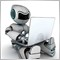| 12 Nov 2016 at 23:53
Congrats for the article. I'm studying signals based on Fibonacci channels, something like https://www.mql5.com/en/code/585
Do you know any similar signal? Thanks.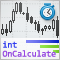How to quickly develop and debug a trading strategy in MetaTrader 5

Scalping automatic systems are rightfully regarded the pinnacle of algorithmic trading, but at the same time their code is the most difficult to write. In this article we will show how to build strategies based on analysis of incoming ticks using the built-in debugging tools and visual testing. Developing rules for entry and exit often require years of manual trading. But with the help of MetaTrader 5, you can quickly test any such strategy on real history.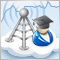How to copy signals using an EA by your rules?

When you subscribe to signals, such situation may occur: your trade account has a leverage of 1:100, the provider has a leverage of 1:500 and trades using the minimal lot, and your trade balances are virtually equal — but the copy ratio will comprise only 10% to 15%. This article describes how to increase the copy rate in such cases.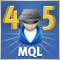Cross-Platform Expert Advisor: Orders

MetaTrader 4 and MetaTrader 5 uses different conventions in processing trade requests. This article discusses the possibility of using a class object that can be used to represent the trades processed by the server, in order for a cross-platform expert advisor to further work on them, regardless of the version of the trading platform and mode being used.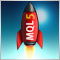MQL5 vs QLUA - Why trading operations in MQL5 are up to 28 times faster?

Have you ever wondered how quickly your order is delivered to the exchange, how fast it is executed, and how much time your terminal needs in order to receive the operation result? We have prepared a comparison of trading operation execution speed, because no one has ever measured these values using applications in MQL5 and QLUA.